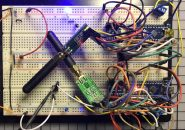## Arduino预处理器指令教程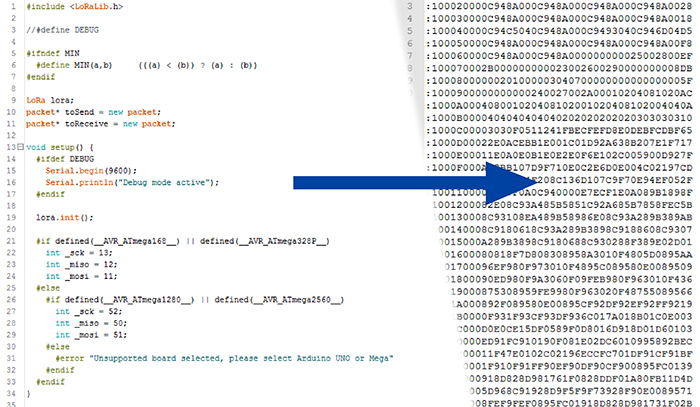• Arduino UNO

• Arduino IDE

## 按“上传”之后

1. Arduino IDE执行了一种名为“语法检查”的操作，以确保您编写的程序是真正的C/C++源代码。此时，如果发生函数拼写错误或忘记分号，那么编译就会停止。
2. 语法检查之后，Arduino IDE会启动另一个名为preprocessor（预处理器）的程序。这是一个非常简单的程序，如果文件是C/C++源代码，它不会怎么样。我们稍后会详细讨论这一步骤。那么现在我们假设结果是一个名为“扩展源代码”的文件——一个文本文件。
3. 然后，该扩展源代码被移交给另一个名为compiler（编译器）的程序。该编译器（在Arduino IDE中是avr-gcc）接收文本源，并生成汇编文件。汇编一种人类可读的低级编程语言，但是更接近机器代码——适用于特定处理器的指令。这里就是您编写程序之前必须选择正确Arduino板的原因——不同的开发板具有不同的处理器，而处理器又具有不同的指令集。
4. 处理您Arduino程序下一个的系统程序叫做assembler（汇编程序）。该程序会生成一个“目标文件”。该文件主要是机器代码，但也可以包含针对其他目标文件对象的“引用”。这允许Arduino IDE“预编译”一些编写Arduino程序时会始终用到的库，从而使整个过程更快。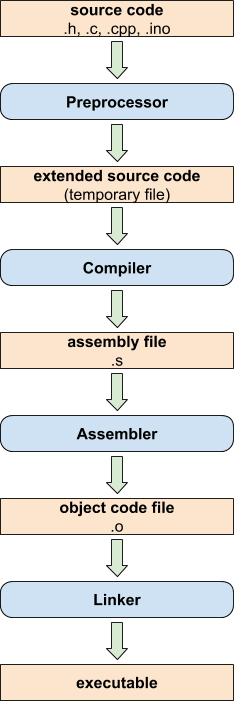## 添加额外代码：#include 指令

``````#include <file>
``````

``````#include "file"
``````

``````#include <ExampleLibrary.h>

void setup() {

}

#include <ExampleLibrary.h>

void loop() {

}
``````

``````//This is an example library

int a = 0;

//End of example library
``````

“ExampleLibrary.h”的内容如下所示。同样，除了一个整数变量之外，没有多少内容。那么当我们编译这个Arduino程序时会发生什么呢？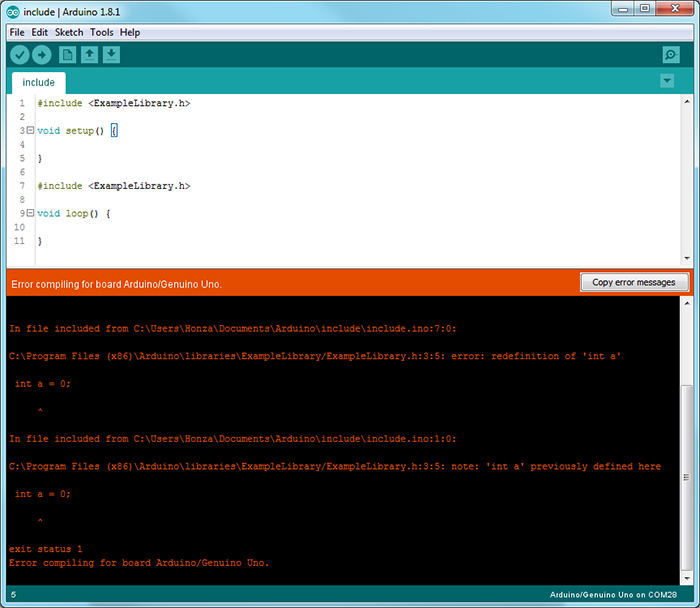``````//This is an example  library

int a = 0;

//End of example library

void setup() {

}

//This is an example  library

int a = 0;

//End of example library

void loop() {

}
``````

``````#ifndef _EXAMPLE_LIBRARY_H
#define _EXAMPLE_LIBRARY_H

//This is an example  library

int a = 0;

//End of example library

#endif
``````

## 定义事物：#define 指令

``````#define macro_name macro_body
``````

``````#define X 10
``````

``````int Y = 10;
``````

``````int Y = X;
#define X 10
int Z = X;

void setup() {

}

void loop() {

}
``````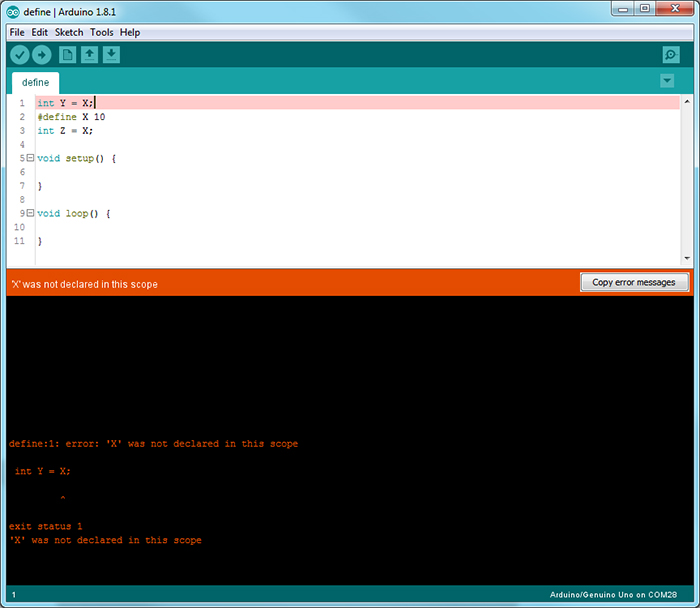``````int Y = X;
int Z = 10;

void setup() {

}

void loop() {

}
``````

``````int min(int a, int b) {
if(a < b) {
return(a);
}
return(b);
}
``````

``````int min(int a, int b) {
return((a < b) ? a : b);
}
``````

``````#ifndef MIN
#define MIN(A, B)     (((A) < (B)) ? (A) : (B))
#endif
``````

``````#ifndef MULTIPLY
#define MULTIPLY(A, B)     A * B
#endif

//some code...

int result = MULTIPLY(2 - 0, 3);
``````

``````int result = 2 - 0 * 3;
``````

``````#ifndef MULTIPLY
#define MULTIPLY(A, B)     ((A) * (B))
#endif
``````

## 条件编译：#if指令

``````#if expression

//compile this code

#elif different_expression

//compile this different code

#else

//compile this entirely different code

#endif
``````

``````#ifndef macro_name

//compile this code if macro_name does not exist

#endif
``````

``````#ifdef macro_name

//compile this code if macro_name exists

#endif
``````

``````#if defined(__AVR_ATmega168__) || defined(__AVR_ATmega328P__)

//this will compile for Arduino UNO, Pro and older boards
int _sck = 13;
int _miso = 12;
int _mosi = 11;

#elif defined(__AVR_ATmega1280__) || defined(__AVR_ATmega2560__)

//this will compile for Arduino Mega
int _sck = 52;
int _miso = 50;
int _mosi = 51;

#endif
``````

## 提供反馈：#warning 和 #error 指令

``````#warning "message"
``````

``````#error "message"
``````

``````#if defined(__AVR_ATmega168__) || defined(__AVR_ATmega328P__)

//this will compile for Arduino UNO, Pro and older boards
int _sck = 13;
int _miso = 12;
int _mosi = 11;

#elif defined(__AVR_ATmega1280__) || defined(__AVR_ATmega2560__)

//this will compile for Arduino Mega
int _sck = 52;
int _miso = 50;
int _mosi = 51;

#else

#error “Unsupported board selected!”

#endif
``````

## 结论

1. 编写库时，请务必将其放在 #ifndef – #define – #endif结构中。这个结构我们已经见过多次了。这可能会为您省去一些麻烦。定义类似函数的宏时同样应该这样做。
2. 编写代码时，应确保程序易于移植到其他Arduino板上。相信我，未雨绸缪要比出现不兼容问题之后再想法解决要容易得多。
3. 分而治之！几个较小的文件总比一个1000多行的大文件要好得多。##### Jan Gromes

Jan目前在布尔诺理工大学学习电气工程。他拥有多年使用Arduino和其他微控制器构建项目的经验。他的特殊兴趣在于机器人系统的机械设计。

### 相关文章

1.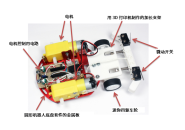#### 在最后一刻停下来！用Arduino和距离传感器制作小鸡赛车！（第3篇•最终篇）

2.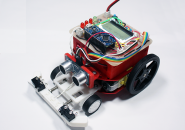#### 在最后一刻停下来！用Arduino和距离传感器制作小鸡赛车！（第2篇）

3.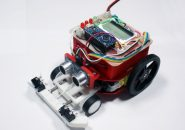#### 在最后一刻停下来！用Arduino和距离传感器制作小鸡赛车！（第1篇）

4.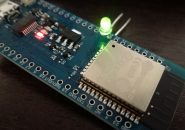#### 通过Arduino使用ESP-WROOM-32（环境搭建和配置-LED闪烁篇）

5.#### 通过Arduino使用ESP-WROOM-32（Bluetooth-BLE连接篇）

6.#### 六种可以用于扩展物联网应用的DIY项目

7.#### 使用Arduino的五大DIY无人机项目

8.#### 从电动滑板到无人机：5个DIY项目来提升您的电子技能

9.